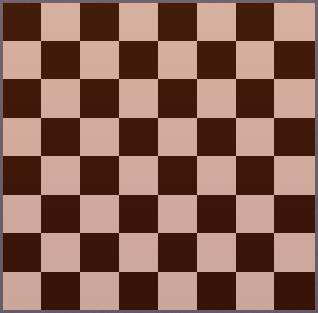# Chess Board

Probability Level 2In permutations and combinations , one of the problems that appear frequently is asking for the total number of squares on 8 x 8 chessboards. Can you tell what the answer is?

As an extension, can you find a formula to count the number of squares in N x M rectangle ?

×